## Asian Journal of Biomedical and Pharmaceutical Sciences

Review Article - Asian Journal of Biomedical and Pharmaceutical Sciences (2019) Volume 9, Issue 66

## Analysis of lossless compression techniques time-frequency-based in ECG signal compression.

Hamed Hakkak*, Mahdi Azarnoosh

Department of Biomedical Engineering, Mashhad Branch, Islamic Azad University, Mashhad, Iran

*Corresponding Author:
Hamed Hakkak Department of Biomedical
Engineering Mashhad Branch
Islamic Azad University Mashhad, Iran
E-mail: [email protected]

Accepted Date: January 2, 2019

Citation: Hakkak H et.al. Development and validation of an Enzyme Linked Immunosorbent Assay (ELISA) for gentamicin quantification in dried blood spot samples. Asian J Biomed Pharmaceut Sci. 2019;9(66):16-25.

### Abstract

Technological advances introduce different methods for telecardiology. Telecardiology includes many applications and is one of the long-standing medical fields that have grown very well. In telecardiology, a very high amount of ECG data is recorded. Therefore, to compress electrocardiogram data (ECG), there is a need for an efficient technique and lossless compression. The compression of ECG data reduces the storage needs for a more efficient cardiological system and for analyzing and diagnosing the condition of the heart. In this paper, ECG signal data compression techniques are analyzed using the MIT-BIH database and then compared with the Apnea-ECG and Challenge 2017 Training bases. During the study, some of the various techniques of frequency analysis, range and time are widely used, such as run-time coding, AZTEC, Spin-Coding, FFT, DCT, DST, SAPA/FAN and DCT-II, where DCT and SAPA/FAN have the best compression performance compared to other methods.

## Keywords

ECG data compression techniques, SAPA/FAN, DCT, FFT, CR, PRD, ECG signal.

## Introduction

Biological signals are derived from biological processes. This process is very complex and dynamic. Biomedical signals are usually a function of time. To describe the biological signals, Rick's solution is quasi-periodic, depending on how we need it and how accurate it is. In the alternating pseudo-signal, the form is repeated approximately at specified intervals. Transient signals occur only once over time. The waveform of such signals is indefinite and can only be described using statistical concepts [1-6]. Depending on the biological process, random signals are divided into static and non-stationary types. In static random signals, the statistical characteristics of the signal do not change over time. If the biological process generating a random signal in a given condition is the accidental generated signal will be invalid. For example, an ECG is a non-stop random signal (Figures 1 and 2).

A computerized system of biological signal processing requires a huge amount of data. It is very difficult to store and process these signals. We need a way to reduce the amount of data storage, and this should be done keeping their critical clinical content in order to rebuild the signal. Compressing information reduces the transport time and the storage space and reduces the memory capacity in portable systems, in other words, increases the number of channels to be transferred and broadens the bandwidth. Compressing information is used in many fields as well as in medical science . The latest advances in biomedical signal processing, information and communication technology, have brought new metrics to the medical world. Recently, several studies and techniques have been developed to compress the signal.

ECG signal compression is an emerging issue as well as an investigation that has many benefits in health care, such as minimal storage space, to at least utilize channel bandwidth in transmission . In today's world despite significant advances in medicine, heart disease is still a major concern and is one of the common causes of death in patients. Given the importance of this issue, today, in advanced societies, attention is increasingly paid to electrocardiographic systems. The task of these systems is to receive a patient's heart signal and send it to the hospital for a quick assessment of the patient's condition. An ECG is an important parameter that measures patient health and reports if there is a failure. Electronic health care emphasizes the effectiveness of the signal processing preamplifier unit, so that wireless data transmission is more efficient . Algorithms with a better compression ratio and less damage to data in a reconstructed signal are required . Compression of ECG data reduces the storage needs for a more effective cardiological system for cardiac analysis and diagnosis .

This study conducted a survey of various types of ECG data compression techniques. It discusses the various techniques proposed in studies for compressing an ECG signal and provides comparative studies of these techniques. The MITBIH ECG Compression Test database has been used in this study and a pre-processing is required. The database contains 168 short electrical records (20.48 per second) that are used to create various challenges in ECG compression, especially for time-frequency compression techniques. We have used 35 data to examine the methods.

The results obtained from this work are influenced by the data base of the database. Given the fact that the purpose of finding the best methods in time and frequency domain using the parameters mentioned, perhaps the use of a database does not provide comprehensive results, but one should take the following points: 1. This database is in the number A lot of previous related work is used and is well-suited. 2. In any case, there is a limitation on the use of resources, and it must be remedied by techniques. 3. In the end, the method works on several databases other than The MIT-BIH ECG Compression. And one can mention the Apnea-ECG Database and Challenge 2017 Training Set Databases, which solves some of the problems.

The Rest of the paper is structured as follows: in Section 2 is summarized. Section 3 introduces proposed compression techniques, including run-time coding, AZTEC, Spin-Coding, FFT, DCT, DST, SAPA/FAN and DCT-II, which are described in the program. Section 4 describes the performance evaluation and comparison of proposed methods. Section 5 shows the experimental results and shows the comparison using suggested methods. Finally, in Section 6, discussions, conclusions and conclusions about the work done are dealt with.

## Related Work

A compression method (ECG) is presented based on a combination of empirical analysis (EMD) and wavelet transform. The ECG signal can be decomposed into a set of intrinsic functions by EMD. The proposed method compresses the inherent state functions into two groups and compresses each group separately to fully utilize the data properties. The first group can be completely uncorrupted by its errors with a reconstruction error, while the second group decomposes by converting the violin. A one-dimensional Wavelet transform does not require much information in the location, while extreme coding of one or two IMFs from high-frequency sectors captures the ECG signal with many points. By choosing the right threshold and using Run Length and Hoffman Coding, the proposed method demonstrates competitive performance compared to other compressions for compression of the ECG. The algorithm does not require prior knowledge to be easily implemented .

ECG compression is based on empirical analysis (EMD). Using the purification process in EMD, the function of proper connections of spin in from the successive meanings together with the first intrinsic functions can be fully constructive of the ECG signal; the proposed method is used with a maximum average for compression. According to the process of screening the IMF in EMD, moderate excess is used in compression and rebuilding. With the optimal choice of mean and dead space quantification and the use of Run Length and Huffman Coding for further compression, several recording of experimental results associated with the MIT-BIH arrhythmia database indicates that proposed methods have better compression results than ECG compressors. The performance of the reconstruction is significantly better than compression based on the encoding of the IMF cover, as well as better compression results than DWT and DCT compression .

Electrocardiogram (ECG) compression technique (LPC) based on linear flow filters and threshold functions is investigated and improved. In this method, the ECG signal is taken from the MITBIH arrhythmic database and compression is obtained by distributing the ECG signal linearity coefficients to the linear predictive encoder. Then various thresholds such as hardness, soft, combination, soft-soft and thresholding techniques are used. At the end of the receiver, the reverse method applies this method and the ECG's original signal is restored. The simulation results show that the 'db4' Wavelet filter improves the results compared to other Wavelet filters. Thresholding is better than other threshold techniques in terms of reliability parameters, such as signal-to-noise ratio (SNR), percent root-mean-square difference (PRD), maximum error (ME), and compression ratio (CR) .

Electronic health care emphasizes the effectiveness of the signal processing preamplifier unit, so that wireless data transmission is more efficient. In order to reduce the power of the system in the long-term acquisition of ECG, it describes a patient-centered QRS diagnostic process for monitoring arrhythmias. This part of the relevant ECG section, for example, specifies the RR distance between the QRS complex for the evaluation of heart rate changes. This processor is made up of a fourth-order optical wavelet transform followed by a modular detection stage. This is done through an FIR symmetric filter, while the second involves a number of extraction steps: zero crossing detection, peak detection (derived-zero), threshold setting, and two devices with finite state for implementing decision rules. The CMOS-0.35 processor produces 300 GHz only 0.83, comparable to previous ones. In system experiments, input data is transmitted from analogue to digital 10-bit SAR, while output data is transmitted through a unique wireless transmitter (TI CC2500), which can be configured by the processor for different modes: 1) The result of the diagnosis of QRS, 2) ECG raw data, or 3) Both. Validation of all records from the MIT-BIH arithmetic database has a sensitivity of 99.31% and predictability of 99.70% .

An improved method for compression of electrocardiogram signals (ECG) is based on correlation coefficient, signal coefficient and main component (PC) for ECG signal. For this purpose, the two-dimensional matrix of the ECG signal is generated based on time correlation between the pulse and further compression using PC extraction. BP correlation increases compression efficiency. A detailed analysis is provided for ten signals containing various rhythms, morphology and anomalies with the database (MIT-BIH). Compact data is obtained using PCA based on the reduction of the characteristic of the correlated data matrix. The overall compression and reconstruction efficiency depends on the choice of the feature and the correlation coefficient. However, the compression and reconstruction efficiency is affected by the correlation coefficient of the beat to the ECG signal. The average CR is obtained with an acceptable quality of compact data of 6: 1. Therefore, this technique can be effectively used to compress ECG signals, and has various morphologies. The effectiveness of proposed method with several features such as percent root-mean-square difference, compression ratio, signalto- noise ratio and correlation have been investigated. Experimental results have shown that this method is very efficient for compression and is suitable for different telecardiogram applications .

A hierarchical tree pattern (SPIHT) pooling is an efficient program that is widely used in electrocardiogram (ECG) compression systems. Based on a bit display of quantum wells, it suggests a modified SPIHT algorithm for fast encoding without loss of compression. A bit-plane is constructed by a tree data structure consisting of two primary tree types. Initial number refers to the number of input sampled data. The bitplane data programming process can be considered as a synthesis that decides according to different rules. The rules of the convention will be simplified to a logical level in the form of a flagship design. Using the MIT-BIH arithmetic database, the experimental results show that the proposed algorithm can reduce coding scheduling by comparing with the traditional SPIHT algorithm with a cost of 0.28% increase in the 64.35% decrease bit. The proposed algorithm is simple, regular and modular .

A compression algorithm for data (ECG) for remote monitoring of cardiac patients from rural areas has been reported based on a combination of two methods of encoding with discrete cosine transformation. The proposed technique provides a good compression ratio (CR) with low PRD values. To evaluate the performance of the proposed algorithm, 48 ECG signal records have been taken from the MIT-BIH arithmetic database. Each ECG signal record is 1-minute-long and sampled at 360 Hz sampling frequency. The ECG signal noise has been removed using the Savitzky-Golay filter. To convert a signal from a time domain to a frequency domain, a discrete cosine transform is used to use the signal energy to reduce the frequency factor. After normalizing and converting conversion factors, the signal is coded using dual encryption techniques, which include run length and Hoffman coding. The dual encryption technique is compressed without any loss of information. The proposed algorithm averages CR, PRD, quality score, normal percentage RMS, RMS and SNR errors of 11.49, 3.43, 3.82, 5.51, 0.012 and 60.11 db .

The development of new signal processing technology offers higher requirements for processor performance. Due to the bottlenecks, the computing performance of general processors is limited and cannot meet the program requirements. Highperformance and low-power FPGAs that have recently been willing to research for a heterogeneous computing platform with a processor. The pulse compression algorithm is widely used in signal processing, which includes a number of floatingpoint calculations, and the processing impact largely depends on the performance of the processor. Based on Open Computing Language (Open CL), we first evaluated the FFT fast Fourier transform from different sample sizes in the Arria10 FPGA, and FPGA gained 33.5 times the performance improvement compared to the DSP C6678 in the processing of various FFT samples. Then a pulsed compression processing of 4K × 8K size is examined using a central channel. The results show that the main calculations on the Arria10 FPGA through Open CL are approximately ten times faster than the C6678 DSP for pulse compression processing at 4K × 8K .

The compression of the QRS series provides an ECG signal with a hybrid technique. This method uses both cascade and parallel DCT and DWT combinations. Method/statistical analysis: the QRS series is an important part of the ECG signal used by doctors to diagnose. The transmission of QRS requires less memory and complexity compared to the full electromagnetic signal. This method uses both cascade and parallel DCT and DWT combinations. Functional measurements such as PRD (root mean square error) and CR (compression ratio) are used to validate the results. Findings from the MIT-BIH ECG database are used for study. The threshold technique applies to both cascading and parallel systems. The cascade system with CR has less memory. Both cascade and parallel systems show good quality repair with low PRD .

Electrocardiogram (ECG) monitoring today plays an important role in helping patients with heart disease. Compressing ECG data is important for portable solutions, reducing storage requirements and low power consumption. Different types of ECG compression algorithms and their implementation are presented in the past. Choosing the flexible ECG technique is appropriate considerations such as storage conditions and the use of communication links. There is a need for more energy and the need for computational complexity in the ECG compression algorithm. In this way, ECG compression techniques tailored to the needs given are still a major challenge from the point of view of implementation. Examining different methods of ECG compression implementation in the literature can be used to identify the appropriate technique for specific needs .

A wavelet-based hybrid electrocardiogram (ECG) signal compression technique is proposed. Firstly, in order to fully utilize the two correlations of heartbeat signals, 1-D ECG signal are segmented and aligned to a 2-D data arrays. Secondly, 2-D wavelet transform is applied to the constructed 2-D data array. Thirdly, the set partitioning hierarchical trees (SPIHT) method and the vector quantization (VQ) method are modified, according to the individual characteristic of different coefficient sub-band and the similarity between the sub-bands. Finally, a hybrid compression method of the modified SPIHT and VQ is employed to the wavelet coefficients. Records selected from the MIT/BIH arrhythmia database are tested. The experimental results show that the proposed method is suitable for various morphologies of ECG signal, and that it achieves high compression ratio with the characteristic features well preserved .

## Compression Methods

Data compression techniques can increase storage capacity and transfer speeds, and reduce the hardware requirements for storage and bandwidth requirements for the network. We make compression through a computer program. The computer program uses various formulas and techniques to reduce the overall volume of recorded data. The compressed signal is reconstructed with some errors. Another classification is based on the techniques applied to compression and can be categorized as follows:

Run length coding compression

One way to reduce the sampling frequency is to send samples and time between samples. Instead of sending the samples, the time between them is sent, but if the send is also ascending or descending, the signal can be restored. This method is simple and good compression. It can be implemented with a digital counter. The synchronization is used for code blocks. This method improves with application of predictive coding and uneven threshold levels .

The run length coding is used to code normal and transparent ECG signal conversion coefficients. Normalized and rounded coefficients, long tasks from data points are similar and can be saved using run length. For example:

X=[2 2 2 2 0 0 0 0 0 4 4 4 3 3]

Can be represented using run length encoding Y=[2 4 0 5 4 3 3 2]

X contains 14 elements and the encoded signal contains 8 elements. Therefore, the run encoding improves the use of duplicate data and compression performance .

Run Length Encryption is an algorithm used to compress data. This compression method is unlucky and is suitable for compressing the types of signals regardless of its information content, but the data content affects the amount of compression obtained by RLE. In this method, the physical size of the repetition of characters is reduced by encryption. For example: a string [AAAAAAAAAAAAAAAAbbbb] can be used as [A4b5] using the RLE encoded . Compression is performed using two QMF channel filters and a RLE-based hybrid algorithm. ECG compression is performed in the following steps:

Step 1 Get an ECG signal, Step 2. The ECG signal obtained is divided into a different frequency band, step 3. Thresholds, Step 4. Apply Run-length encryption to improve compression ratio, step 5. Apply Run-length decryption, step 6. Combine the signals with a synthesis filter bank .

Since the run-length coding is not lost, loyalty to image restoration is guaranteed. It can effectively reduce the volume of data and have less computational complexity .

Amplitude Zone Time Epoch Coding (AZTEC) compression

The AZTEC algorithm has been developed for pre-processing the real-time vital signal for rhythm analysis. This popular algorithm reduces data for ECG monitors. The AZTEC algorithm converts raw ECG sample points into Fatah. The magnitude and length of each plateau are stored for reconstruction. Although the AZTEC method is suitable for compression with CR, the repair error is not clinically acceptable [7,14].

This method of signaling the ECG turns into different slopes and horizontal lines called Plateaus. In this Plateaus, the zero interpolator is used. In this compression technique, information is stored containing the signal in the form of the sample domain and the length of the received signal through the received signals. When the length is less than three, a slope is formed. This technique is not suitable for compression of ECG data because it is not useful in resolving the compression of the signal in the form of reset steps. The pathway for diagnosis by the cardiologist, this is more dominant in the P and T sections of the signals. The following steps are needed to implement this technique 

• An ECG signal, T (t) is loaded.

• Next, we must calculate for i ϵ {1 ... N-2}, if the result is with (T (ti)-T (ti+2))> the threshold value, then we must have T (ti+1) in the above expressions. N is the total number of samples in the signal taken before compression.

• In the method of rebuilding and squeezing the signal, an interpolation technique called Interpolation Cubic Spline will be applied.

Spinal coding compression

The Quadratic Spline Wavelet Transform (QSWT) is used with dense support as the motherboard. This is the first derivative of smooth operation. QSWT applies to QRS detection. Currently, this method holds the highest detection rates compared to other methods. The dense support feature enables the FIR filter to be less trapped. The Fourier transform, which is characterized by its frequency response, is as follows: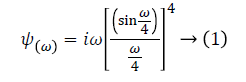The corresponding filter coefficients are;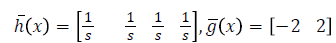The output signal, called the Wavelet coefficients, is related to the smooth derivative of the input signal. It can be said that the triangular waveform is similar to the peak to the maximum modulus pair (positive-max-negative-minimum-pair). The zero crossing point corresponds to the maximum peak-to-peak pair .

The timeline transforms the time and frequency information simultaneously. Different mother liquids such as mother Wavelet, Db4 Wavelet andWavelet Spline are applied to ECG data for the detection of QRS complexes. The results are obtained for eachWavelet, which has more accurate and faster results than the rest. The main advantage of this type of Wavelet is to detect less time for the ECG signal in the long run. The results show that it is possible to calculate peak R with good accuracy. The precision of the Cubic split wavelet is greater .

Fast Fourier Transform (FFT) compression

TheDiscrete Fourier Transform (DFT) or Fast Fourier Transform (FFT) is often used to identify peaks and in turn is used to identify the gene sequence. Frequency domain conversion is performed from the time domain gene sequence using the Fast Fourier Transform Algorithm (FFT). This algorithm requires few resources compared to conventional FFT methods . Fast Fourier transform (FFT) converts a signal from time domain to frequency domain rapidly. Then, the frequency having the maximum amplitude between 1 and 2 Hz is chosen, FFT gives accurate results .

By using the Fourier transform, we can obtain the frequency of the given signal domain . The FFT limit is that it cannot provide the exact location information of the frequency component at the time.A fast Fourier transform has been used to identify ECG signal characteristics .

FFT compression algorithm: 

• Divide ECG components into three components x, y, z.

• Frequency and time between two samples are found.

• Finding the FFT of the ECG signal and checking the FFT (before compression)=0, increasing the counter A if it is between 25 and 25, and index=0.

• Examining FFT coefficients (after compression)=0, increasing the counter B.

• FFT calculation and de-compression display, error.

Discrete Cosine Transform (DCT) compression

The Discrete Cosine transform (DCT) has been widely used due to energy compression and good correlation for compression of ECG data. This conversion needs a reduction power, where the main signal can be reconstructed with just a few coefficients . DCT is a limited sequence of data points in the form of a cosine function that oscillates at different frequencies. The DCT is like a DFT that converts the signal from the spatial domain to the frequency domain . DCT compressed signal information can be restored to a limited number of DCT coefficients. DCT is a discrete signal f (u), u=0, 1, …, N-1 is defined as follows:  DCT constraints have greater distortion in the reconstruction signal.

Basically, in all proposed algorithms for DCT, the amount of data used by the transfer of a subset of conversion factors that carry more information is used. Some solutions use advanced techniques for pre/post processing of the DCT coefficient .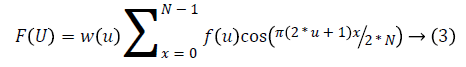The DCT represents a signal as a summation of different frequency sizes. This condition shows a different boundary and is often used in signal and image processing to compress lost data. DCT has "energy intensive properties" and provides a direct link. DCT information in the signal can retrieve DCT data .

DCT compression algorithm: 

• The components of the ECG are three components of x, y, z.

• Frequency and time between two samples are found.

• Finding the DCT of the ECG signal and checking the DCT coefficients (before compression)=0, increasing the counter A if it is between 0.2-0.22 and 0.22 and index=0.

• Increase B count

• Compute DCT reverse and compression display, error.

A discrete cosine is presented to indicate the efficiency of the ECG signal in the frequency space .

Discrete Sine Transform (DST) compression

The Discrete Sine transform (DST) is a Fourier transform that is similar to a Discrete Fourier transform (DFT), but occurs using a completely realistic matrix. Like any Fourier variation, a discrete sinusoidal transform (DSTs) has the expression of a function or signal based on the sum of the sinuses with frequencies and domains. Like a Discrete Fourier Transform (DFT), a DST works on a function in a limited number of discrete dots. The obvious distinction between DST and DFT is that it uses only sinusoidal functions, while Fourier uses both the cousin and the sinus. However, this remarkable difference is only the result of a deeper distinction: DST shows different boundary conditions than DFT and other relevant changes .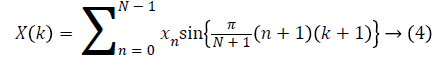DST compression algorithm: 

• Construct the ECG components into three components: x, y, z.

• Frequency and time between two samples are found.

• Find DST Signal ECG and DST check.

• The coefficients (before compression)=0, the increase of the counter A if it is between 15 and 15 and index=0.

• Review of DST (after compression)=0, increase of B.

• Compute DST Reverse and Displays Compression, Error.

Discrete Cosine Transform-II (DCT-II) compression

The most common type of Discrete Cosine transformation is type II. DCT-II is typically used as the actual conversion, using the following equation:The DCT-II can be viewed as a special kind of Discrete Fourier Transform (DFT) with real inputs. This view is positive because it means that any FFT algorithm for DFT immediately leads to a quick-matching algorithm for DCT-II simply by excluding the expansion operation .

DCT-II compression algorithm: 

• Distribute the x sequence in the sequential blocks Nbi=0, 1, ¼ .., Nb-1, each with Lb sample.

• Calculate DCT for each block.

• Quantization of DCT coefficients.

• Un encoded encoding of quantized DCT coefficients.

SAPA/FAN algorithm

The function of this algorithm is that the critical points are identified with regard to the error value, which, of course, determines the value of this error by the user. Obviously, the more the user gets the error rate, the more the number of samples is selected, and as a result, the accuracy of the reconstruction of the signal and the result of the reconstructed signal will be more similar.

Scan-Along Polygonal Approximation Techniques (SAPAs) and split scans into the length of a priority algorithm with two degrees of freedom (FOI-2DF) for compression of the ECG. The FOI-2DF method runs without saving all the actual data points between the last and the last point .

Performance and Comparison Performance Parameters

Compression is a subject-oriented process. None of the compression algorithm cannot claim to be optimal in all conditions. Compressor operation is fully customizable. In other words, the designer of the algorithm cannot invent the nature, properties, and behavior of the signal to be compressed from the algorithm itself to provide an appropriate algorithm.

In the past, many algorithms have been used to compress ECG data. The compression function of these algorithms can be evaluated using the following performance parameters :

Compression ratio (CR)

CR The ratio of the original data to the compressed data regardless of account factors such as bandwidth, sampling frequency, original data accuracy, word length, compression parameters, reconstruction error threshold, database size, line selection and noise level. This means that the higher CR, the smaller the size of the compressed file . Provides information compression ratio on the degree to which blank data compression methods are removed . The higher the compression rate, the less number of bits needed to store or transfer data [7,22,23].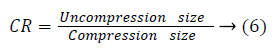Compression Rate (CRC)

The compression ratio is known as compression strength to measure the decrease in data generated by the compression algorithm.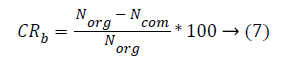Percentage Square Difference (PRD)

This measure evaluates the distortion between the original signal and the reconstruction. As defined by the ratio of the main signal range and the compressed signal range. The compressed ratio provides information about the degree to which blank data compression methods are deleted. The highest compression ratio is the less number of bits needed to store or transfer data. The compression value is measured by CR and the distortion between the original signal and the PRD is restored. The data compression algorithm should demonstrate acceptable loyalty when accessing a high CR. That the PRD reflects the loyalty of reconstruction; its rise in value is actually unpleasant [6,22,24,25].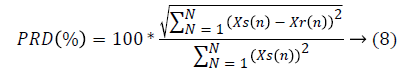Where N is the no of data samples, Xs is the original and Xr is the reconstructed signal.

Quality Score (QS)

This is the normal PRD version that is independent of the X signal value and is obtained using the equation below .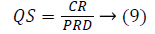Average of Root Mean Square error (RMS)

This coefficient of instability in the reconstructed signal is given according to the initial signal and is given using the equation below [7,14,24].Signal to Noise Ratio (SNR)

Measuring the amount of noise energy by compression on a db scale and using the equation below [7,14,24].Correlation coefficient

The CoC value for the ECG signal should reach 1. CoC is calculated as follows: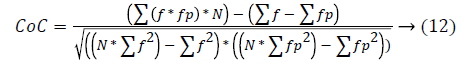Correlation coefficient (CC) is used as an indicator for evaluating the effective loss of information after compression of data .

Time to run the algorithm (T)

The duration of the algorithm for each data, which is the mean runtime for each data group. That time is measured by the computer system below. The proposed compression methods discussed in the previous section are implemented using MATLAB software (i.e., version 9, R-2016a) package installed on Windows 10 platform (i.e., Intel Core I7 processor, 3.5 GHz, 8 GB RAM) for the analysis of ECG signals.

Evaluation of the Results of Different Methods of Compression of the ECG Signal

The non-fixed line and transmission line interference are the most important noises and can strongly affect the analysis of the ECG signal. The non-firm line is from breathing 0.15 to 0.3 hertz. The transmission line interference is a centralized noise band of 60 Hz with a bandwidth of less than 1 Hz. The ECG signals were pre-processed through high-frequency noise filtering, unsecured line-up and transmission line interference, and thus the signal strength increase. The ECG signal is 0.5 Hz less than the low interference and 50 to 60 Hz AC, which is removed using the morphology filter as below [19,20].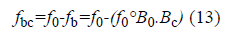f0 is the main ECG signal with noise components, fbc the ECG signal without lacing the base line and B0.Bc the structural elements provided in the ECG .

In this study, the MIT-BIH ECG Compression Test database was used after pre-processing. The database contains 168 short electrical records (20.48 per second) selected to create various challenges for ECG compressions, especially for timefrequency compression techniques. We have used methods for reviewing. Experimental results reported from multiple papers on a number of MIT-BIH records show better performance in existing data .

In Figure 3, the average of the values obtained from each evaluation criterion for all methods is shown to indicate the degree of variation among different registers.

Figure 3: Chart of the changes of the methods examined in the article, in addition to the evaluation parameters.

## Discussion and Conclusion

Compression on the basis of run length encoding, with proper operation in Compression Ratio (CR) and Compression Rate (CRC) parameters, indicates that it is smaller than the other methods of measuring the size of the compressed file and the greater the compression ratio [28-30]. Compression methods by Amplitude Zone Time Epoch Coding (AZTEC) and compression based on the spline coding did not have a good performance in almost any of them and are not suitable for compressing the ECG signal. Compression methods based on Fast Fourier Transform (FFT), Discrete Cosine Transform (DCT) and Discrete Sine Transform (DST) proper operation in the parameters of the Percent Mean Square Difference (PRD), Quality Score (QS), Root Square Error (RMS), indicating that the transformation-based methods have a low distortion between the original and the reconstructed signal and the high compression ratio and proper stability in the signal reconstructed with the initial signal . Compression methods based on Discrete Cosine Transform II (DCT-II) and SAPA/FAN have higher Compression Rate (CRC) and Signalto- Noise Ratio (SNR) values, indicating that the amount of noise is less than other methods and the amount of compression are acceptable. In Figure 4, the compression methods studied in this work have been analyzed with the evaluation parameters.

Figure 4: The values obtained from CD, CRC, PRD, QS, RMSE, Corrcoef, SNRR, T parameters in compression methods.

According to the results obtained from the previous section, methods can be prioritized according to the evaluation criteria, that is, one should see which criterion is more important for the user and choose the method based on it .

In this paper, the comparison of time-frequency, time-domain and frequency-based compression methods have been done on the MIT-BIH ECG Compression Test database. Figure 4 and Table 1 shows the comparative results of compression methods. Table 2 summaries the evaluation results of compress signal ratios for each parameter.

(RLE) (AZTEC) (spline) (DCT) (DST) (FFT) (DCT2) (SAPAFAN)
CR 1.4725 1.0314 0.9996 1.0524 1.0685 1.0129 0.9714 1.6969
CRC 24.067 3.0753 0 5.0195 6.4459 1.3125 22.5391 41.0296
PRD 5.5403 44.991 23.956 0.0274 0.0413 0.0268 12.9886 9.2204
QS 0.301 0.0233 0.2735 41.893 28.3442 42.6148 0.4399 0.1967
RMSE 5.9755 182.65 26.4342 0.0286 0.0429 0.0278 9.1253 9.8335
SNRR 1.0068 0.5426 0.9995 1 0.9999 1 1.017 1.0649
Corrcoef 0.9972 -0.0203 0.9288 1 1 1 0.9911 0.9928
T 0.0652 0.1348 0.0326 0.0402 0.0411 0.042 1.0469 0.0335

Table 1. Compare compression methods.

Method
CR Run length, SAPA/FAN
CRC Run Length Encoding, SAPA/FAN, Discrete Cosine Transform II -
PRD Fast Fourier Transform (FFT), Discrete Cosine Transform (DCT), Discrete Sine Transform (DST)
QS Fast Fourier Transform (FFT), Discrete Cosine Transform (DCT), Discrete Sine Transform (DST)
RMSE Fast Fourier Transform (FFT), Discrete Cosine Transform (DCT), Discrete Sine Transform (DST)
SNRR SAPA/FAN, Discrete cosine transform II -
Corrcoef Fast Fourier Transform (FFT), Discrete Cosine Transform (DCT), Discrete Sine Transform (DST)
T Spline coding, SAPA/FAN

Table 2. The best way to compress signal ratios for each parameter.

The method (AZTEC) and the coding of the spinel violin were not well presented in general. The SAPA/FAN method and the discrete cosine transformation (DCT) method and FFT are relatively better in general .

For future work, checking the rundown encoding compression methods, AZTEC and is in various conversion domains (DCT, FFT, DST, DWT), which aims to identify the best domain for the compression process.